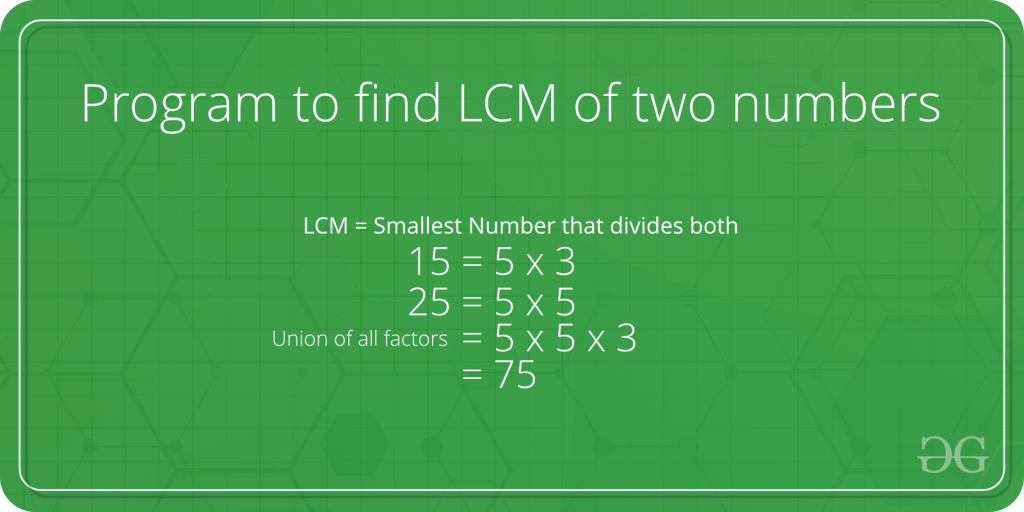# Java Program to Find LCM of Two Numbers

• Difficulty Level : Hard
• Last Updated : 13 Jul, 2022

LCM (i.e. Least Common Multiple) is the largest of the two stated numbers that can be divided by both the given numbers.Example:

`LCM of 15 and 25 is 75, and LCM of 3 and 7 is 21.`

Therefore, firstly find all the prime factors of both the stated numbers, then find the union of all those factors that are present in both the given numbers. And at last, return the product of elements which are in union.

Below given formula for finding the LCM of two numbers ‘u’ and ‘v’ gives an efficient solution.

```u x v = LCM(u, v) * GCD (u, v)
LCM(u, v) = (u x v) / GCD(u, v)```

Note: GCD is the greatest common divisor.

## Java

 `// Java program to find LCM of two numbers.``class` `gfg {``    ``// Gcd of u and v using recursive method``    ``static` `int` `GCD(``int` `u, ``int` `v)``    ``{``        ``if` `(u == ``0``)``            ``return` `v;``        ``return` `GCD(v % u, u);``    ``}` `    ``// LCM of two numbers``    ``static` `int` `LCM(``int` `u, ``int` `v)``    ``{``        ``return` `(u / GCD(u, v)) * v;``    ``}` `    ``// The Driver method``    ``public` `static` `void` `main(String[] args)``    ``{``        ``int` `u = ``25``, v = ``15``;``        ``System.out.println(``"LCM of "` `+ u + ``" and "` `+ v``                           ``+ ``" is "` `+ LCM(u, v));``    ``}``}`

Output

`LCM of 25 and 15 is 75`

Time Complexity: O(log(min(a,b))

Auxiliary Space: O(log(min(a,b))

Similarly, you can find the LCM of any two given numbers.

My Personal Notes arrow_drop_up